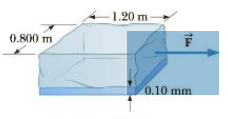Chapter 9, Problem 53P

Chapter
Section
Textbook Problem

The block of ice (temperature 0°C) shown in Figure P9.53 is drawn over a level surface lubricated by a layer of water 0.10 mm thick. Determine the magnitude of the force F → needed to pull the block with a constant speed of 0.50 m/s. At 0°C, the viscosity of water has the value η = 1.79 × 10−3 N· s/m2.Figure P9.53

To determine
The magnitude of the force F needed to pull the block with a constant speed.

Explanation
The definition of the coefficient of viscosity is η=Fd/Av and this expression is rearranged for F=ηAv/d .

Given info: The viscosity of water is 1.79×103Ns/m2 , thickness of water is 0.10mm , sides of the ice block is 1.20m and 0.800m , and the speed of the block is 0.50m/s .

The formula for the magnitude of the force needed to pull the block with a constant speed is,

F=ηAvd

η is viscosity of water.

A is area of the block.

v is speed of the block.

d is thickness of the block.

Substitute 1.79×103Ns/m2 for η , 1.20m×0

Still sussing out bartleby?

Check out a sample textbook solution.

See a sample solution

The Solution to Your Study Problems

Bartleby provides explanations to thousands of textbook problems written by our experts, many with advanced degrees!

Get Started